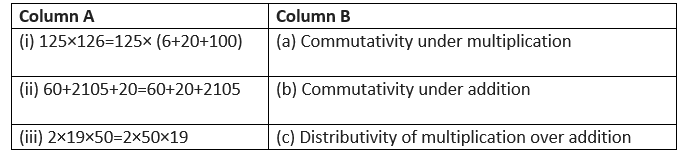# Practice test for Class 6 Maths whole Numbers -2

In this page we have Practice test for Class 6 Maths whole Numbers . Hope you like them and do not forget to like , social share and comment at the end of the page.
Topic of Properties of Whole numbers is deleted from this chapter as per rationalized syllabus.

## Multiple Choice Questions

Question 1
What is the predecessor of 3452?
a. 3455
b. 3451
c. 3453
d. 3452
Question 2
What is the successor of 978?
a. 977
b. 979
c. 980
d. 981
Question 3
What is the correct sign for this?
430 ___ 403
a. >
b. <
c. =
d. none of these
Question 4
What is the correct sign for this?
12345 _____ 45678
a. >
b. <
c. =
d. none of these
Question 5
What is the correct sign for this?
460 ___ 406
a. >
b. <
c. =
d. none of these
Question 6
What is value of 7 – 5?
a. 1
b. 2
c. 3
d. 4
Question 7
Which whole number has no predecessor?
a. 0
b. 1
c. 2
d. none of these
Question 8
Which of the following will not represent zero?
a. 1 + 0
b. 0 × 0
c. 0/11
d. (110-110)/2

## Fill in the blanks

Question 9
a. If the product of two whole numbers is zero, then ______ of them will be zero.
b. Every natural number except _____ has a predecessor.
c. If we add the number _____ to the collection of natural numbers, we get the collection of whole numbers.
d. All natural numbers are __________ numbers.
e. 900 is the successor of _____.
f. A number remains unchanged when multiplied to ______.
Question 10
Fill in the blanks
(a) ____ × 13 = 13 × 24
(b) Whole numbers are closed under_______ and ________ operation.
(c) Division by ______ is not defined.
(d) _______ is the identity for multiplication.
(e) If ______ is added to a number, the sum will remain the same. Hence ______is called the __________ in the whole numbers.

## Long type Question

Question 10
Every classroom in a Dehradun school contains 32 students. How many students would 5 classrooms hold?

Question 11.
Find the sum by suitable rearrangement: $237 + 108 + 163$.
Question 12
Find the product by suitable rearrangement: $4 \times 4768 \times 50$.
Question 13
Find the product using suitable properties: $854 \times 103$.

## Match the column

Question 15
Match the following:2. b
3. a
4. b
5. a
6. b
7. a
8. a
9.
a. one
b. 1
c. 0
d. whole
e. 899
f. 1

10.
a. 24
c. zero
d. 1
e. zero ,zero , additive identity
11. $5 \times 32 = 160$
12. $237 + 108 + 163 = 237 + 163 + 108 =400 + 108=508$
13. $4 \times 4768 \times 50 =4 \times 50 \times 4768= 200 \times 4768 =953600$
14. $854 \times 103 = 854 \times (100 + 3) = 85400 + 2562= 87962$
15. i - > c
ii -> b
iii -> a

• Notes
• Assignments
• NCERT Solutions### Practice Question

Question 1 What is $\frac {1}{2} + \frac {3}{4}$ ?
A)$\frac {5}{4}$
B)$\frac {1}{4}$
C)$1$
D)$\frac {4}{5}$
Question 2 Pinhole camera produces an ?
A)An erect and small image
B)an Inverted and small image
C)An inverted and enlarged image
D)None of the above